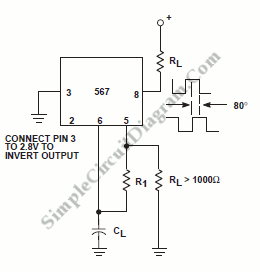# Oscillator With Quadrature Output Using 567 IC

Oscillator circuit is an electronic circuit that produce a periodic signal. The term quadrature refers to a fourth (1/4) phase shift of full wave cycle (1/4 of 360′ = 90′). In square wave oscillator, a quadrature simply means a 1/4 period phase shift. Here is a schematic diagram of such circuit:This circuit uses a 567 tone decoder circuit, which is  capable of generating an oscillation as well as detecting/decoding a frequency/tone. The component values calculation to get certain frequency operation is similar to general 567 IC based oscillator, f = 1/(1.1xR1xC1) [Circuit’s schematic diagram source: Philips Semiconductors Application Notes]